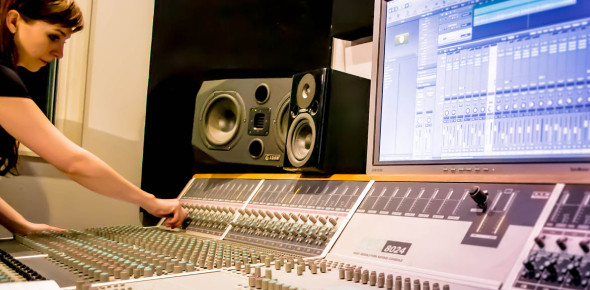# Audio Engineering - Digital Audio

15 Questions | Total Attempts: 1304SettingsThe primary duty of most sound technicians is to record or reproduce sound using audio equipment. However, they may also be responsible for setting up audio and mixing board equipment for sporting events or artistic productions. Test out what you know about digital audio by taking up the comprehensive test below. All the best!

• 1.
Which of the following is an accurate description of Bit Depth
• A.

Defines the minimum dynamic range of a track

• B.

States how many samples are taken per second

• C.

States how much data passes per second

• D.

States the number of different values each sample can be

• 2.
If the Bit Depth is 16bit, which of the following statements is true
• A.

There are 16 samples per second

• B.

There are 16 different dynamic levels each sample can be

• C.

Each sample can be any value of representable by 16 1's and 0's

• D.

Bit depth relates to the frequency response

• 3.
If the bit depth was 8, how many different amplitude levels can be recorded
• A.

8

• B.

16

• C.

128

• D.

256

• 4.
Which of the following make up the settings for a Red-Book Audio CD (pick 4)
• A.

Sample Rate = 44,100hz

• B.

Sample Rate = 48khz

• C.

Bit Depth = 128kb/s

• D.

Bit Depth = 16

• E.

Maximum Length = 74 minutes

• F.

Maximum Number of Tracks = 99

• G.

Minimum Number of Tracks = 3

• H.

Minimum Length = 5minutes

• I.

All tracks in WAV format

• 5.
If the sample rate is 48khz which of the following statement is true
• A.

Each sample can be 48,000 different values

• B.

The highest recordable frequency is 48khz

• C.

The recorded wave form is sample 48,000 times per second

• D.

The track is sampled 48 times

• 6.
Which of these statements is NOT true:
• A.

Increasing the sample rate increase the range of recordable frequencies

• B.

Increasing the sample rate increases the amount of data required to store the file

• C.

Increasing the sample rate increases the amount of time each wave form is sampled

• D.

Increasing the sample rate increases the range of dynamic levels available

• 7.
Which of the following statements is an accurate description of Aliasing
• A.

Occurs as a result of the bit depth being too low

• B.

A "ghost" low frequency is recorded when a frequency above the sample rate is recorded

• C.

A ghost high frequency is recorded when a frequency below half the sample rate

• D.

Can be avoided by using a high-pass filter

• 8.
An anti-aliasing filter can be created using which of the following EQ filters
• A.

Band-Pass

• B.

Low Pass

• C.

High Pass

• D.

High Shelf

• E.

Low Shelf

• 9.
Which of the following statements is true regarding in relation to the Niquist Theory:
• A.

To avoid aliasing, the highest frequency must be half the sample rate

• B.

To avoid aliasing, the highest frequency must be double the sample rate

• C.

To record the wave form accurately, the highest frequency must be half the sample rate

• D.

To record the wave form accurately, the highest frequency must be double the sample rate

• 10.
What is the minimum amount of times that a wave form must be sampled in order to be replayed at the correct frequency accuracy
• A.

1

• B.

2

• C.

3

• D.

4

• 11.
If the sample rate is 48khz, what is the highest frequency which could be recorded without aliasing occuring.
• A.

96khz

• B.

48khz

• C.

24khz

• D.

24hz

• 12.
Niquist theory applies to what kind of recording:
• A.

Digital Audio

• B.

MIDI

• C.

Analogue Audio

• D.

Kinetic Energy

• 13.
Which of the following signals paths would be an accurate possible chain of events for Analogue to Digital Conversion
• A.

Analogue Signal > High Pass Filter > A/D Converter > Digital Signal

• B.

Digital Signal > D/A Converter > Analogue Signal > Amp > Speaker > Audible Signal

• C.

Digital Signal > A/D Converter > Analogue Signal > Amp > Speaker > Audible Signal

• D.

Audible Signal > Microphone > Analogue Signal > Low Pass Filter > A/D Converter > Digital Signal

• 14.
Which of the following statements is NOT true regarding an A/D Converter running at 48khz, 16bit
• A.

Turns an analogue signal into a string of 1's and 0's

• B.

Measures the amplitude of the wave form 48,000 times per second and stores it as a 16bit word

• C.

Measures the frequency of the waveform 48,000 times per second

• D.

It generates 768000 bits per second

• 15.
If the bit depth is 16, however many different levels of amplitude can be recorded
• A.

16

• B.

(2 x 16 =) 32

• C.

(16 to the power of 2 = 16 x 16 =) 256

• D.

(2 to the power of 16 = 2 x 2 x 2 x 2 x 2 x 2 x 2 x 2 x 2 x 2 x 2 x 2 x 2 x 2 x 2 x 2 =) 65, 536Back to top